IntroductionIntroduction# Volume of Cones and Pyramids

INTRO
Volume tells us the amount of space there is inside a given 3D shape. It’s kind of like the 3D version of area. To find the volume of a cone or pyramid, we need to multiply the area of the base by the height, and then divide by 3.This formula is very similar to the formula for the volume of a cylinder and prism. The only difference is that there’s one additional step...dividing by 3!
CALCULATOR

## Volume of Cones and Pyramids Calculator

What does your shape look like?

#### Step 1. Imagine & identify the base.

We can think of the cone as a cake. The base is the bottom layer, a circle.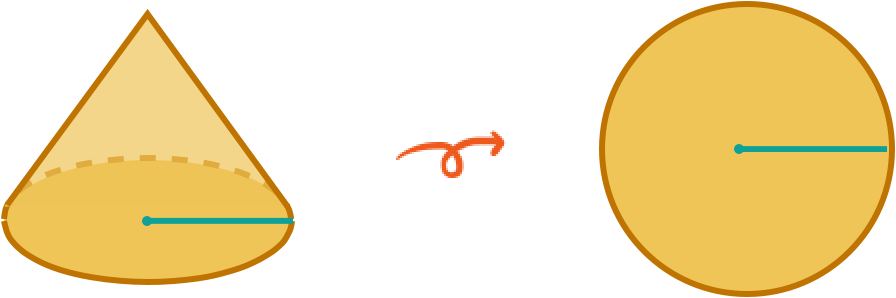KEY STEPS

## How to Find the Volume of Cylinders & Prisms

Shape:

### Step 1. Imagine & identify the base.

We can think of the cone as a cake with layers shaped as circles. The base is the bottom layer, a circle.

### Step 2. Calculate the area of the base.

We start by calculating the area of the base circle.

### Step 3. Multiply the area of the base by the height (H) of the cone.

We can think of this step as stacking up layers of the cake base until you reach the desired height.

### Step 4. Divide by 3.

We can think of this step as taking a piece off the left and right sides, leaving us with of the cake.
LESSON
Volume of Cones & Pyramids

## Volume of Cones & Pyramids

Like cylinders and prisms, the two main things we need to know in order to solve for the volume of a cone and pyramid are the area of the base and the height of the 3D shape.The formula for the base area depends on the shape of the base.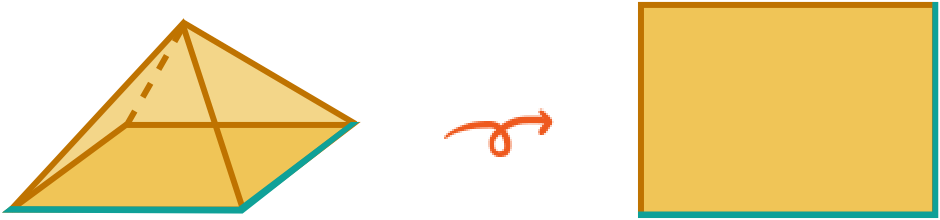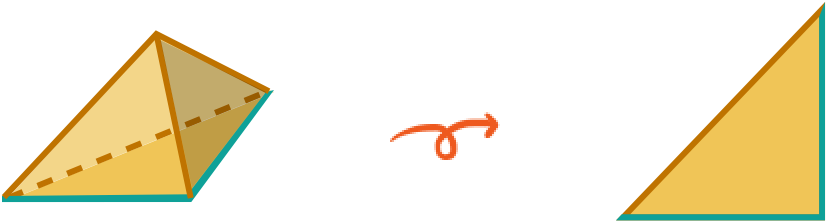Once we have base area, we multiply it by the height, and then divide by 3! 👌🏾👌🏽👌🏻
PRACTICE
Volume of Cones & Pyramids

## Practice: Volume of Cones & Pyramids

Question 1 of 6: Find the volume of this cone: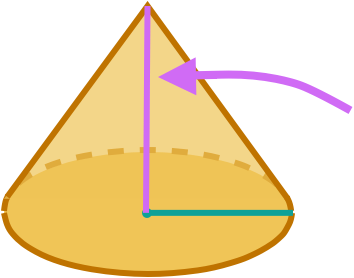### Step 1. Imagine & identify the base.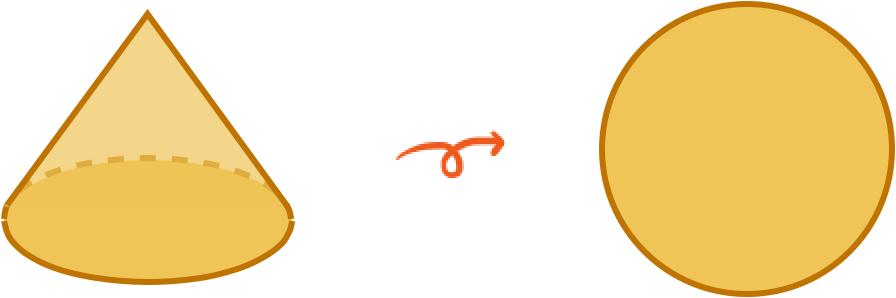The base of a cone is a  .

CONCLUSION
Nice work, look at you go! Thanks for checking out this lesson ☺️🙏. Where to next?Leave Feedback